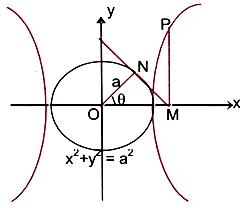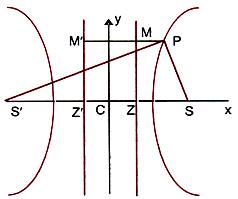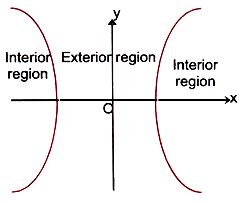×#### Thank you for registering.

One of our academic counsellors will contact you within 1 working day.

Click to Chat

1800-1023-196

+91-120-4616500

CART 0

• 0

MY CART (5)

Use Coupon: CART20 and get 20% off on all online Study Material

ITEM
DETAILS
MRP
DISCOUNT
FINAL PRICE
Total Price: Rs.

There are no items in this cart.
Continue Shopping```Propositions of a Hyperbola

Table of Content

What is a Hyperbola?

Diameter of a Hyperbola

Conjugate Diameters

Latus Rectum

Director Circle of a Hyperbola

Asymptotes

Relation between Focal Distances

Relative Position of a Point with respect to the Hyperbola

Related Resources

What is a Hyperbola?A conic whose eccentricity is greater than unity is called a hyperbola. A hyperbola may be defined to be the locus of a point whose distance from a fixed point called focus and from the fixed line called directrix is a constant called ‘e’ and in this case, ‘e’ > 1.

The standard equation of a hyperbola is x2/a2 – y2/b2 = 1 where b2 = a2(e2 - 1).

The general equation of hyperbola, whose focus is point (h, k), directrix is lx + my + n = 0 and eccentricity ‘e’ is given by

(x-h)2 + (y-k)2 = e2(lx + my + n)2/(l2 + m2)

All the important terms related to hyperbola have been discussed in the previous sections. We discuss here some of the propositions and important properties of hyperbola:

We can express the coordinate of a point of the hyperbola x2/a2 – y2/b2 = 1 in terms of a single parameter, say θ.In the figure given above, OM = a sec θ and PM = b tan θ. Thus any point on the curve, in parametric form is x = a sec θ, y = b tan θ.

In other words, (a sec θ, b tan θ) is a point on the hyperbola for all values of θ. The point (a sec θ, b tan θ) is briefly called the point ‘θ’.

Diameter of a Hyperbola

The locus of the middle point of a system of parallel chords of a hyperbola is called its diameter.

The equation of the diameter is y = b2x/(a2m), where m is the slope of the system of parallel chords.

Conjugate Diameters

Two diameters of a hyperbola which bisect chords parallel to each other are called conjugate diameters.

∴ The diameters y = mx and y = m1x of the hyperbola x2/a2 – y2/b2 = 1 are conjugate if mm1 = b2/a2.

Latus Rectum

The chord of a hyperbola through one of the foci and at right angle to the transverse axis is called the latus rectum.

∴ If 2l be the length of the latus rectum, then the co-ordinates of one of its extremities is (ae, l).

∴ The point (ae, l) lies on the hyperbola x2/a2 – y2/b2, so we have

e2 (l2/b2) = 1

⇒ l2 = b2(e2–1) = b2a2(e2–1)/a2 = b4/a2 (since b2 = a2(e2 – 1)

⇒ l = b2/a2

∴ The length of the latus rectum = 2b2/a2

Director Circle of a Hyperbola

The director circle is the locus of the point of intersection of a pair of perpendicular tangents to a hyperbola.

Equation of the director circle of the hyperbola x2/a2 – y2/b2 = 1 is x2 + y2 = a2 – b2i.e. a circle whose centre is origin and radius is √(a2 – b2).

Note:

If b2 < a2, this circle is real.

If b2 = a2, the radius of the circle is zero, and it reduces to a point circle at the origin. In this case the centre is only point from where tangents at right angle can be drawn to the hyperbola

If b2 > a2, the radius of the circle is imaginary, so that there is no such circle, and so no tangents at right angles can be drawn to the circles.

Watch this Video for more reference

Asymptotes

If the length of the perpendicular from a point on the hyperbola to a straight line tends to zero as the point on the hyperbola tends to infinity along the hyperbola then the straight line is called the asymptote of the hyperbola.

Hence, a line, which is tangent to the hyperbola at infinity, but which is not itself at infinity, is called the asymptote of the curve.

To find the equation of the asymptotes of the hyperbola x2/a2 – y2/b2 = 1:

Let y = mx + c be an asymptotic to the given hyperbola. Then eliminating y, the abscissas of the points of intersection of y = mx + c

or x2(b2 – a2m2) – 2a2mcx – a2(b2 + c2) = 0                 …… (1)

If the line y = mx + c is an asymptote of the hyperbola then it touches the hyperbola at infinity i.e. both the roots of the equation (1) are infinite and for this we must have b2 – a2m2 = 0 and 2a2mc = 0. Hence we get m = + (b/a) and c = 0.

∴ The asymptotes are y = + (b/a) x

Or x2/a2 + y2/b2 = 0

∴ Their combined equation is (x/a + y/b)(x/a – y/b) = 0 or x2/a2 – y2/b2 = 0 which shows that the equation of the asymptote differs from that of the hyperbola in the constant term only. Also the angle between the asymptotes is 2tan–1(b/a).

The lines x2/a2 ± y2/b2 = 0 are also asymptotes to the conjugate hyperbola x2/a2 - y2/b2 = 1.

Key Notes:

The equation of the hyperbola and that of its pair of asymptotes differ by a constant. For example, if S = 0 is the equation of the hyperbola, then the combined equation of the asymptotes is given by S + K = 0. The constant K is obtained from the condition that the equation S + K = 0 represents a pair of lines. Finally the equation of the corresponding conjugate hyperbola is S + 2K = 0.

Any line drawn parallel to the asymptote of the hyperbola would meet the curve only at one point.

When b = a, then the hyperbola is rectangular and its asymptotes are y = ± x which are at right angles.

The asymptotes of the hyperbola and its conjugate are the same.

Asymptotes pass through the centre of the hyperbola and the bisectors of the angles between the asymptotes are the axes of the hyperbola.

They are in fact a tangent to the hyperbola from the centre.

Asymptotes of a hyperbola are the diagonals of the rectangle formed by the lines drawn through the extremities of each axis parallel to the other axis.

A perpendicular drawn from the foci on either asymptote meet it in the same points as the corresponding directrix and the common points of intersection lie on the auxiliary circle.

If a straight line is drawn from any point of the asymptote in such a way that it is perpendicular to the transverse axis, then the product of the segments of this line intercepted between the point and the curve is always equal to the square of the semi conjugate axis.

If the angle between the asymptote of a hyperbola  x2/a2 – y2/b2 = 1 is 2θ, then e = sec θ.

The tangent and normal at any point of a hyperbola bisect the angle between the focal radii.

If a circle intersects a rectangular hyperbola at four points, then the mean value of the points of intersection is the mid point of the line joining the centres of circle and hyperbola.

Relation between Focal Distances

The difference of the focal distances of a point on the hyperbola is constant. PM and PM’ are perpendiculars to the directrices MZ and M’Z’ and PS’ – PS = e(PM’ – PM) = eMM’ = e(2a/e) = 2a = constant.Relative Position of a Point with respect to the Hyperbola

The quantity x12/a2 – y12/b2 = 1 is positive, zero or negative, according as the point (x1, y1) lies within, upon or outside the curve.Illustration:

Tangents are drawn from any point on the hyperbola x2/9 – y2/4 = 1 to the circle x2 + y2 = 9. Find the locus of mid point of the chord of contact. (IIT JEE 2005)

Solution:

Let any point on the hyperbola be (3 sec θ, 2 tan θ).

Therefore, chord of contact of the circle x2 + y2 = 9 with respect to the point (3 sec θ, 2 tan θ) is,

(3 sec θ)x + (2 tan θ)y = 9.  …... (1)

Let (x1, y1) be the mid-point of the chord of contact.

Then the equation of the chord in mid point form is

xx1 + yy1 = x12 + y12  ….. (2)

Since both the above equations are identically equal, so

3 sec θ/x1 = 2 tan θ/y1 = 9 (x12 + y12)

This gives sec θ = 9x1/3(x12 + y12)

and tan θ = 9y1/2(x12 + y12)

Eliminating θ from the above two equations we get

81x12/ 9(x12 + y12)2 – 81y12/4(x12 + y12)2 = 1.

Therefore, the required locus is x2/9 – y2/4 = (x2 + y2)2/ 81.

Related Resources

For getting an idea of the type of questions asked, refer the previous year papers.

To read more, Buy study materials of Hyperbola comprising study notes, revision notes, video lectures, previous year solved questions etc. Also browse for more study materials on Mathematics here.

```### Course Features

• 731 Video Lectures
• Revision Notes
• Previous Year Papers
• Mind Map
• Study Planner
• NCERT Solutions
• Discussion Forum
• Test paper with Video Solution